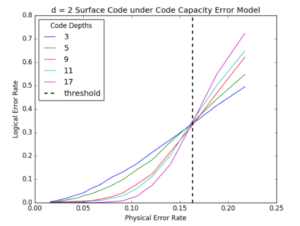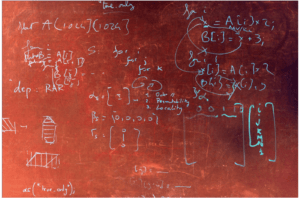##We introduce and analyze a new type of decoding algorithm called General Color Clustering (GCC), based on renormalization group methods, to be used in qudit color codes. The performance of this decoder is analyzed under depolarizing and generalized bit flip noise models, and is used to obtain the first fault-tolerant threshold estimates for qudit 6-6-6 color codes. The proposed decoder is compared with similar decoding schemes for qudit surface codes as well as the current leading qubit decoders for both sets of codes…

## Combinatorial Multigrid for Lattice Quantum ChromodynamicsLattice Quantum Chromodynamics (LQCD) is a numerical approach to understanding the strong nuclear force, in which space and time are discretized into a 4D lattice. Calculating the quark propagator involves inverting sparse, roughly symmetric and diagonally dominant matrices with trillions of entries… Continue reading “Combinatorial Multigrid for Lattice Quantum Chromodynamics”